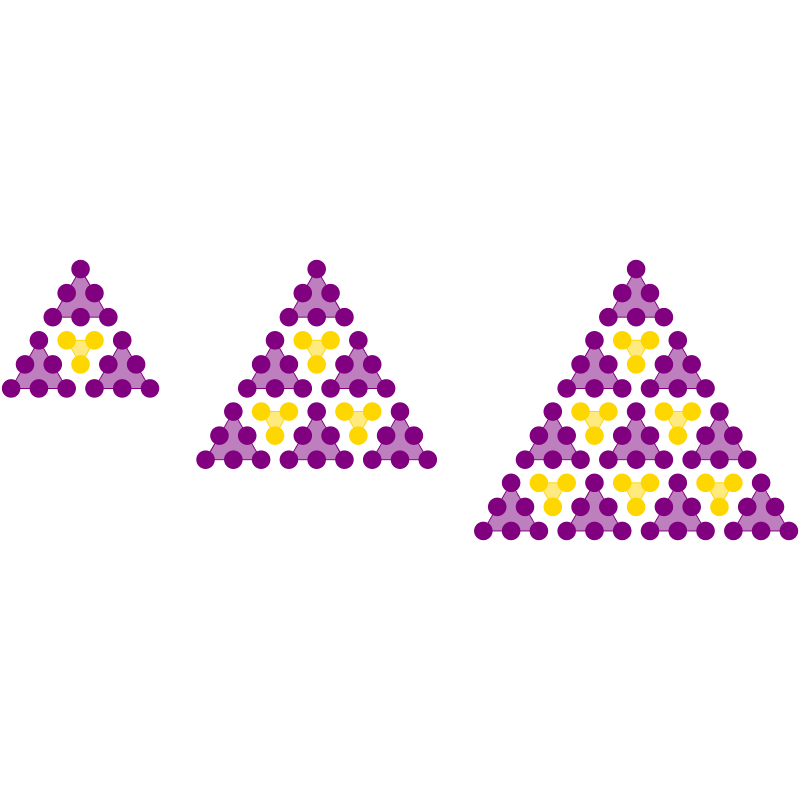A visual proof of an identity involving triangular numbers, created for this blog post, which also contains several other similar proofs.Author: Brent Yorgey
> import Diagrams.Backend.SVG.CmdLine
> {-# LANGUAGE NoMonomorphismRestriction #-}
> import Diagrams.Prelude hiding (dot)
>
> import Data.Colour

Draw a group of dots in a triangular array, all with the same color and backed by a solid-colored triangle to visually group them. Note how the dots are laid out by creating a trail called edge, rotating it 60 degrees, and using decorateTrail to lay out the rows of dots.

> mkTri c n = dots <> (strokeLoop edges # lc c # lw thin # fcA (c withOpacity 0.5))
>   where rows = map (hcat' (with & sep .~ 1 ))
>              . zipWith replicate [n,n-1..1]
>              . repeat
>              $dot c > dots = cat' v (with & sep .~ 3 & catMethod .~ Distrib) rows > v = rotateBy (1/6) unitX > edge = fromOffsets . replicate (n-1)$ unitX # scale 3
>         edges = glueLine (edge <> rotateBy (1/3) edge <> rotateBy (2/3) edge)
>
> dot c = unitCircle
>       # lw none
>       # fc c
>
> rowSpc = height (rotateBy (1/6) \$ strutY 1 :: D V2 Double)

row k n s c draws a row of k size-n triangles with color c, separated by enough space for s dots.

> row k n s c = hcat' (with & sep .~ 1 + 3*s) (replicate k (mkTri c n))

The visual proof, which simply consists in assembling various sub-triangles into a larger triangle, using appropriately transformed and aligned instances of row.

> law4 k n c1 c2 = vcat' (with & sep .~ rowSpc) (map tRow [1..k])
>   where tRow k = (row k n 0 c1 # centerX # alignT)
>                  <>
>                  (row (k-1) (n-1) 1 c2 # reflectY # centerX # alignT)

Finally, create a row of diagrams showing the proof at different sizes.

> exampleRow f = hcat' (with & sep .~ 4) . map f --(alignB . f)
>
> law4Dia = exampleRow law4' [2..4]
>   where law4' k = law4 k 3 purple gold
>
> example = law4Dia # frame 0.2
> main = mainWith (example :: Diagram B)